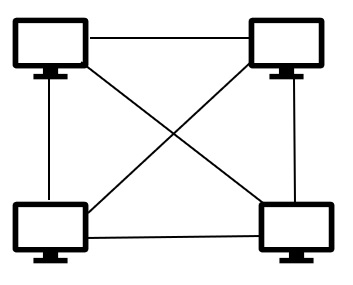# What is a Mesh Topology in Computer Networks?

Topology refers to the arrangement of computer networks. Topology is divided into either physical or logical arrangement. Physical topology refers to the way the computers are connected with each other on a network. Logical topology means the way data is flowing from one computer to another computer.

Now, let see the basic definition of computer network topology, it is the schematic description of network arrangements that are connected through various nodes with the help of lines of connection.

## Types of Network Topology

The different types of network topology are as follows −

• Bus topology
• Ring topology
• STAR topology
• MESH topology
• TREE topology
• Hybrid Topology

Let us understand the concept of MESH topology in computer networks.

## MESH topology

It is a point-to-point connection to all devices. In this arrangement all the network nodes are connected with each other. Generally, for n devices mesh has n(n-1)/2 physical channels.

### Techniques

Mesh topology uses two techniques to transmit data over topology. They are as follows −

• Mesh Topology Routing Technique − In routing, the nodes have routing logic, as per the network requirements. The routing logic directs the data to reach the destination using the shortest distance, or it informs the broken links so that the network will avoid those nodes. The routing helps to reconfigure the failed nodes.

• Mesh Topology Flooding Technique − In flooding, the same data is transmitted to all network nodes. Hence, no routing logic is required here. Moreover, in flooding, the network is robust, and it is not interested to lose data, but it leads to unwanted load over the network.## Types

Now let see the different types of MESH Topology, which are explained below −

• Partial Mesh Topology − Here, the systems are connected the same as mesh topology but some devices are only connected to two or three devices, that is each device is not connected to all devices, that’s why we call it a partial mesh topology.

• Full Mesh Topology − Here, each and every node is connected to each other, so that is the reason we used to call it Full mesh topology.

## Features

The features of MESH Topology are as follows −

• Fully connected.
• Not flexible.

The advantages of MESH topology are as follows −

• Each and every connection can carry its own data load.
• It is robust.
• Fault is identified easily.
• It provides security and privacy.